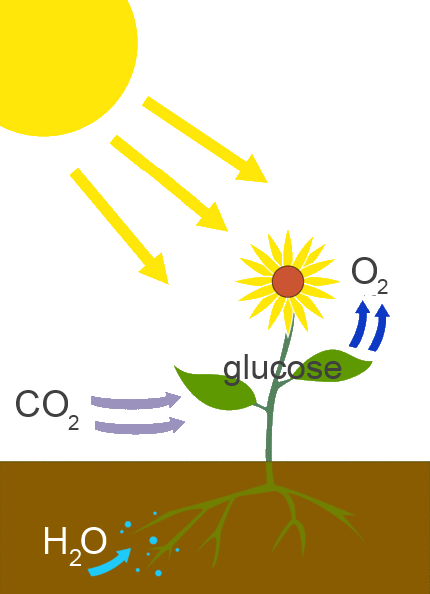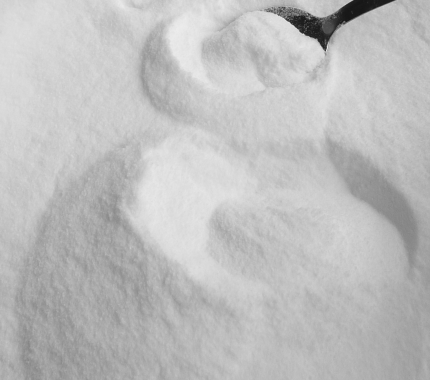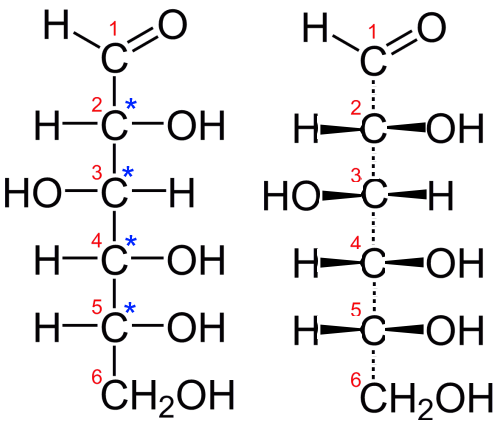Search:

# Glucose

## The origin

Glucose is the main product of photosynthesis. Plants put it in the reserve as such or converted into cellulose, sucrose, wood etc ..Photosynthesis: $6CO_2$ $+$ $6H_2O$ $\rightarrow$ $C_6H_{12}O_6$ $+$ $6O_2$ Carbon dioxide and water $\rightarrow$ glucose and oxygen

In its pure state, glucose is the white solid well-known to athletes and cooks ..## Chemical functions

Glucose: $C_6H_{12}O_6$ $CH_2OH(CHOH)_4CHO$ 5 alcohol functions; one aldehyde function

## Configuration

Glucose has 4 asymmetric carbon atoms. Here (left) its Fischer projection (remember: bonds "horizontal = forward; vertical = backward")The atom $C$ number 2 has its $OH$ group oriented to the right, hence the name D-glucose D-glucose rotates the plane of polarization of polarized light to the right, hence the name D(+)-glucose

## Cyclic form

In aqueous solution, there is a balance between "linear" and "cyclic" formsAs the "linear" form is actually not rigid (free rotation about $C-C$ bonds), the $O$ atom of carbon No. 5 can make a nucleophilic attack on carbon No. 1 followed by the exchange of a $H^+$ ion.

## Exercise

Find the absolute configurations (Kahn-Ingold-Prelog configurations) $R$ or $S$ ) of the $C$ atoms 2,3,4 and 5 in linear glucose !